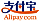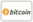Copertina di Harmonized Fractal Dimensional Measure
Titolo del libro:

# Harmonized Fractal Dimensional Measure

Scholars' Press (13.05.2015 )eleggibile a buono
ISBN-13:

### 978-3-639-76410-9

ISBN-10:
3639764102
EAN:
9783639764109
Lingua del libro:
Inglese
Risvolto di copertina:
Herein is proposed a dimensional risk measure based on harmonized fractal behaviour by analysing the probability survival distribution of the returns with aim to quantify the incurred risk, test for coherency and compare the subsequent dimension measures with the harmonized fractal measure (HFM). The advantage of our approach is that this measure is act as maximum entropy to the original fractal measure, adjusts the true fractal measure to give more weight to higher dimension risk events. Here the risk and its capital requirement are harmonized on fractal dimensional measure. It is a much simplified model and could be used as a guide to obtain portfolio selection policies that are nearly as good as the optimal ones from practical concern. Also as a device to determine the likelihood estimators of random variable of real life data set in finance logistics in capturing the dynamic natures of financial markets on tradeoffs between time specific risk, loss occurring at a point in time or dimensions. We have shown here that HFD measure satisfy the condition for subadditive and, translative invariant hence coherent while FDM does not because the covering set are all taken to be equal.
Casa editrice:
Scholars' Press
Sito Web:
http://www.scholars-press.com
Da (autore):
Bright O. Osu, Obiageri E. Ogwo
Numero di pagine:
64
Pubblicato il:
13.05.2015
Giacenza di magazzino:
Disponibile
categoria:
Matematica
Prezzo:
45,90 €
Parole chiave:
Portfolio Selection, HARMONISED FRACTAL, DINENSIONAL MEASURE, Risk Measure### Categorie